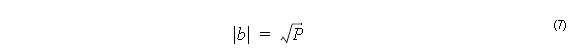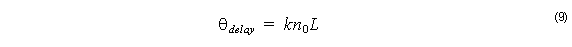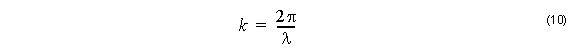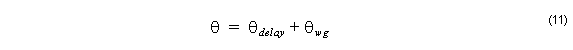The first quantity needed is P , the Power in Output Waveguide. Calculate the electric field amplitude, a complex number b . The modulus of this complex number is just the square root of P ,The real and imaginary parts of b are given by the optical phase θ asThere are two terms contributing to θ , one is from the phase delay due to the length
of the layout regionwheren0  is the reference index, and L is the length of the region, the distance from the input plane to the end of the wafer.

The other angle, θwg , is from the waveguides themselves. You can observe this angle in the output files of an OptiBPM calculation. The total angle needed to complete the calculation is